Home / Blog / Artificial Intelligence / Activation Functions

# Activation Functions

• by Ms. Karnam Shubha
• May 22, 2020
• 569

In Deep Learning the most significant component is the activation function. It helps to determine the output given inputs.

They play a major role in deciding to activate or deactivate the neurons. A nonlinear transformation is applied to the input layer to find accurate results for complex neural networks. It can be used to normalize the input data.

A Neuron basically has two parts in it, integration and activation.

The integration part has the weighted average of input, this value is then passed to the activation function to get an output.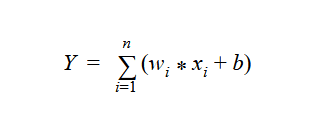## Why Activation Function?

Without an activation function, a neural network will become a linear regression model. But introducing the activation function the neural network will perform a non-linear transformation to the input and will be suitable to solve problems like image classification, sentence prediction, or langue translation.

There are multiple types of activation

• Linear:

A function where its activation is proportional to the input. The output will be the weighted sum of input. Another name for a linear activation function is the identity function.

If a neural network has only a linear activation function then it is just like a linear regression model. It doesn't have the ability to handle complex data with varying parameters.

Using this activation function, it is not possible to achieve gradient descent, as the derivative of this function is constant. Due to which it is not possible to go back and update the weights.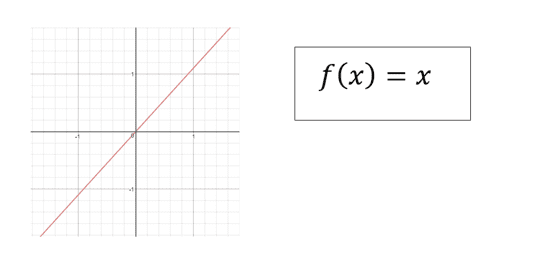• ReLU:

It is called a rectified linear unit, if the value is greater than 0, then it will give away the same value as output. otherwise, it will give 0 as output.

ReLU will help the network to converge quickly. It simply looks like a linear function but it takes care of backpropagation.

However, when the inputs become zero or negative, the gradient of the function becomes zero and hence will not perform the backpropagation operation. This is called "The dying ReLU".

Also, this activation function should be only used in hidden layers of a neural network.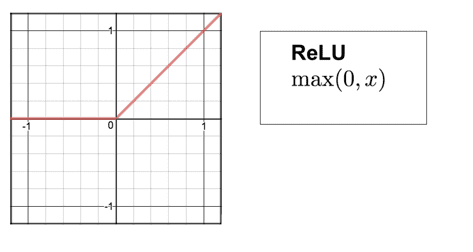• ELU:

The exponential linear unit, it functions similar to the ReLU but, it also considers the negative value. If the value is greater than 0, give away the same value as an output otherwise it gives α (ex-1) where α is a positive constant number.

By looking at the graph, we observe that ELU becomes slowly smooth until its output is equal to -α. To avoid “The dying ReLU" problem ELU is strongly used as an alternative to ReLU.

But there is a problem with ELU, for the values x > 0, it can explode the activation function if the output is ranging from (0, ).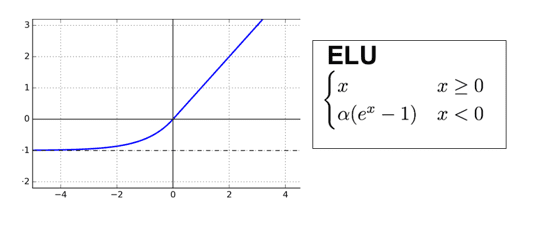• Sigmoid/ Logistic:

A function which takes values as input produces an output within the range of 0 to 1. It is easy to work with as it is continuous, has a fixed range of outputs, and it is differentiable.

The gradient of the sigmoid activation is smooth and can be used as a good classifier. Unlike the linear activation function where the ranges were from (0, ), it has a range (0,1) which will not explode the activation functions.

However, the output values tend to respond less to the changes in inputs giving raise to the vanishing gradient problem. Also, the outputs are not zero centred, it makes the gradient go too far in different directions which are going to make the optimization harder.

The computation process is expensive.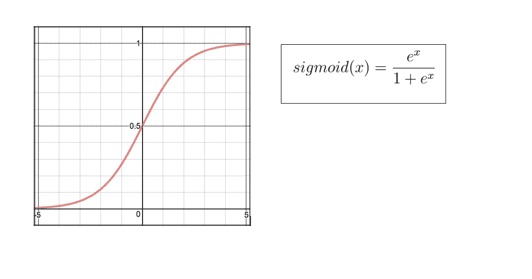• Tanh:

Hyperbolic tangent is an activation function similar to sigmoid but the output values range between -1 to 1. Unlike sigmoid the output of Tanh function is zero centred, therefore Tanh is preferred more than sigmoid.

Tanh performs better than the sigmoid activation functions but it still holds on the vanishing gradient problem.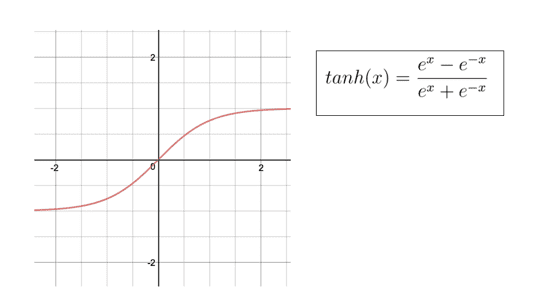• Softmax:

An activation function which will calculate the probabilities of target class overall the target classes. The output of each class is normalized between 0 and 1 and the resulting probability lets know the class of the input.

Sometimes, the names SoftMax and sigmoid will confuse as both the names start with “S” and the values of the outputs are also almost similar(0,1).

One thing to keep in mind about the SoftMax activation function is, it’s been only used in the output layer of the neural network which will solve the multiple class problem.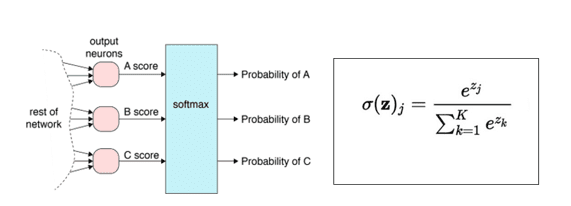• Heaviside step:

It is a unit step function, whose value is 0 for negative numbers and 1 for all positive numbers. It is a discontinuous function which is named after Oliver Heaviside. Since they produce binary outputs, they are very useful for binary classification studies.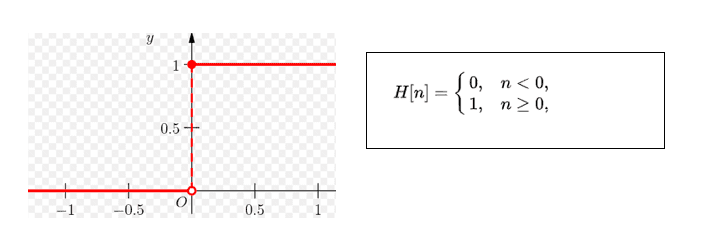• Arctangent:

This activation function is similar to sigmoid and Tanh, it maps the inputs to outputs which range between (-2,2).

Its derivative converges quadratically again 0 for larger values. Whereas, the sigmoid converges exponentially against 0.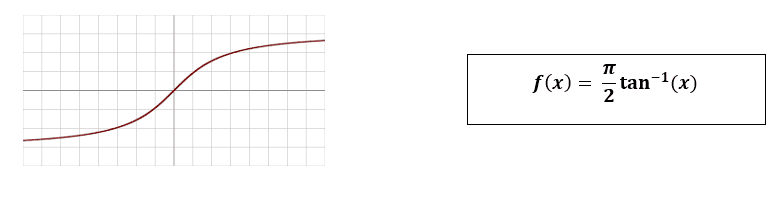• Leaky ReLU:

This activation function is very similar to the ReLU activation function but Leaky ReLU does take the negative values into consideration but it just lowers the magnitude of it.

It is an attempt to fix "The dying ReLU" problem by having a small negative slope. It has a small positive slope in the negative region which allows backpropagation even for negative input values.

Because of its linearity, it can be used to solve the complex classification problems. It somehow lags the sigmoid and Tanh for a few cases. It fails to perform well for negative values,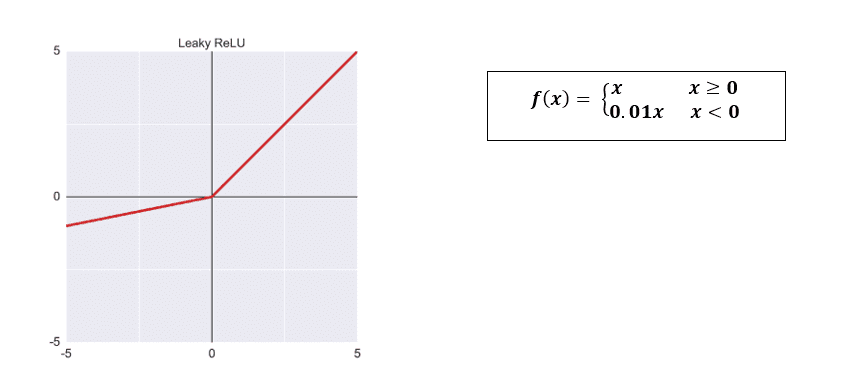• Parametric ReLU:

It is a type of Leaky ReLU where it makes the coefficient of leakage into a parameter.

Leaky Relu gives the negative slope for the negative values, but it is going to behave differently for multiple problems which makes it as one of the disadvantages of this function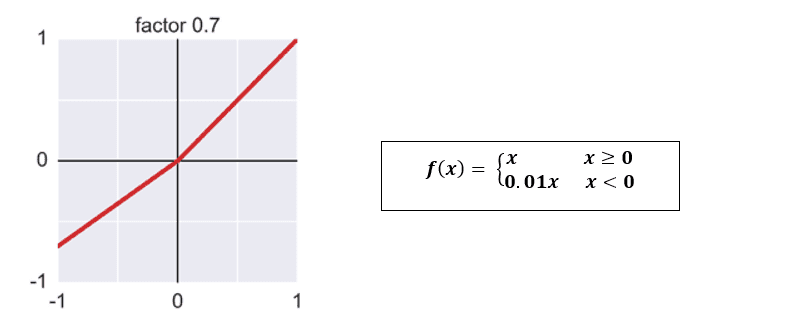• Softplus:

It is a smoothed version of ReLU, both ReLU and Softplus are similar, except near 0 where the softplus is smooth and differentiable.

It was first introduced in 2001, can be used to overcome “The dying ReLU” problem by making itself differentiable and causing less saturation.

The outputs produced by sigmoid and Tanh functions have some range, whereas softplus function produces output in the range (0, ).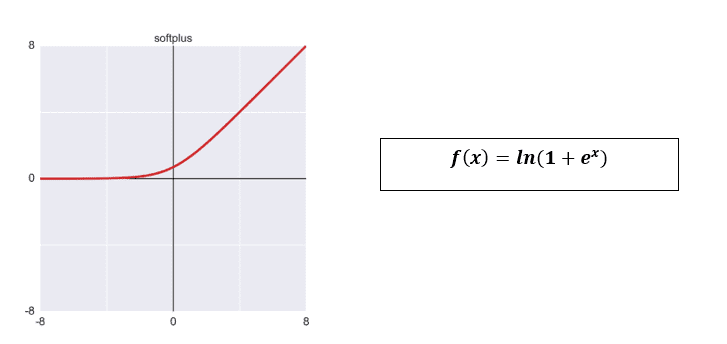• Maxout:

An activation function returns the maximum value among the n values from a linear equation.

It is a combination of ReLU and Leaky ReLU, most of the time it is used along with the drop out technique.

However, the parameters to be learnt by each neuron will be doubled, so it is required to train a lot of parameters.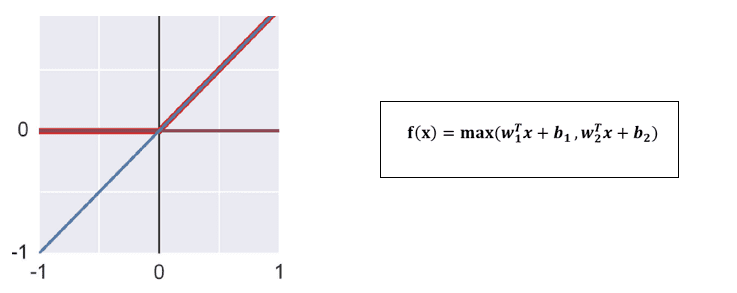• Ramp:

It looks very similar to the sigmoid activation function, it maps the inputs to output over a range (0,1), instead of a smooth curve the ramp will have a sharp curve. It is a truncated version of the linear function.• Shifted ReLU:

It is a variation of ReLU which just moves the bend down and left. It has the flexibility to choose horizontal and vertical shifts.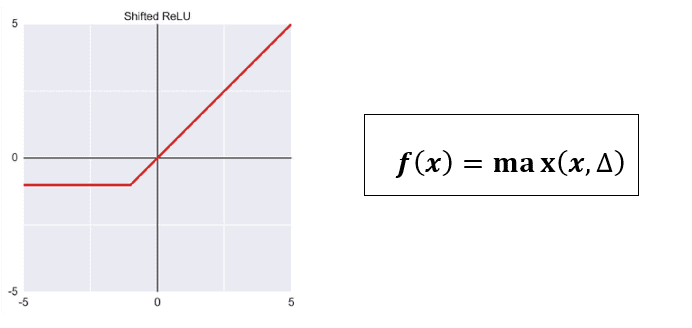• Stair Step:

It gives the output as the floor value of x. Here in this figure, the function gives output if the input is from 0 to just less than 0.2, but then 0.2 if the value is from 0.2 to just less than 0.4 and so on.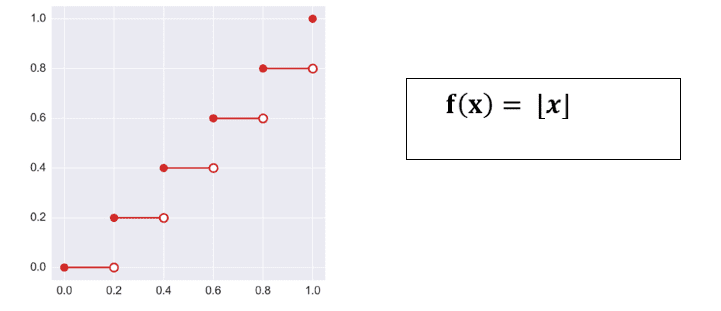• Step:

This is a very basic activation function, a threshold value is decided to give the output. It is used to solve classification and binary class problems.

However,It will not classify the input into other categories.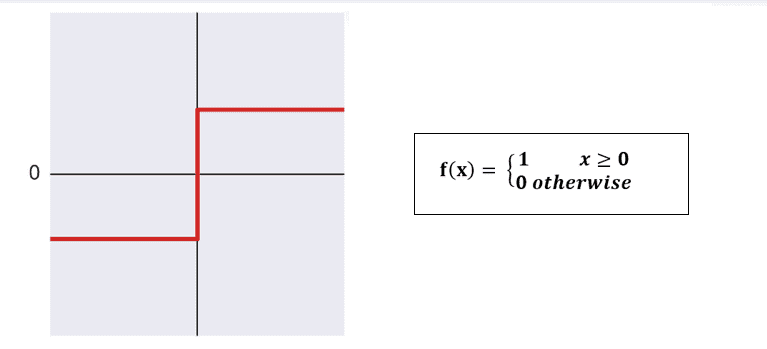• Swish:

It is a combination of ReLU and sigmoid. It is a ReLU with a small, smooth bump just to the left of 0, which then flattens out.

It is discovered by the researchers at GoogleThey claim that this activation function performs better than ReLU with a similar level of computation efficiency.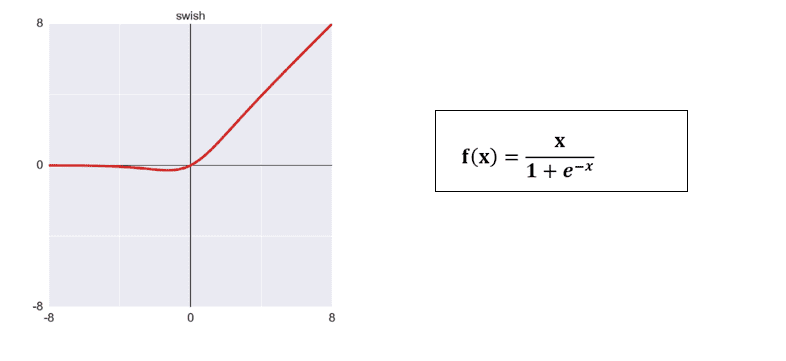Click here to learn Data Science Course, Data Science Course in Hyderabad, Data Science Course in Bangalore

### You may also like...Data Science
August 07, 2020

Data Science has become a leading field of study in recent times owing to its vast use in almost every industry in all parts of the world.Data Science
August 07, 2020

Data Scientists are one of the most sought-after jobs in Malaysia because they are high in demand and also offer enormous pay.Data Science
August 07, 2020

Most of the Data Scientists started their careers as data analysts or statisticians. But the beginning of the escalation in demand and evolution of big data led to the evolution of these roles as well.